cancel
Showing results for
Did you mean:Helper II

## Different result in Excel and Power BI when looking for earliest timestamp by python pandas

I have a list of timestamps. Because there are a few issues, I have to modify some of the timestamps by python.

I have run it in Jupyter and exported the result in Excel. On the other hand, I have tried to run the script in Power BI as well.

Both of them share the same script but found that the result is not 100% identical, some of the results are the same, while some of the results are not the same.

For example, the original timestamp is "08/11/2022 01:35:47", but I have to correct it to "07/11/2022 23:58:34". Because I have to guarantee all items in the same category can be corrected. So I used the following python snippet.

``df['New Hot Time'] = df.groupby("Tractor Cycle Id")["New Hot Time"].transform('min')``

But turns out the "New Hot Time" in Excel and Power BI are not equal to each other.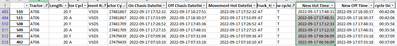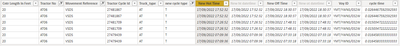I am new to PowerBI, not sure if it is a bug. Hope someone can answer this kind of unusual question. Thank you very much!

1 ACCEPTED SOLUTIONSuper User

If you only run the python code you get the correct result, so instead of first ingesting the file with excel and doing some manipulations in power query and then inserting the python file, if you just run the python code (i adjusted it slightly the code to run directly in the source step of power query) then you get the correct result.    ie in the source step i run the python scriptso when ingest the file i just select  get data and select use python script rather than using excel to get datathis the code i ran in this step

# 'dataset' holds the input data for this script
import pandas as pd

def _truck_type(truck😞
if truck.find("AT") >= 0:
return "AT"
elif truck.find("T") >= 0:
return "MT"
else:
return "XT"

dataset = dataset.sort_values(by=["Tractor No","On Chasis Datetime"], ascending=[True,True])
dataset = dataset.reset_index(drop=True)

dataset["Truck_type"] = dataset["Tractor No"].map(_truck_type)
truck_type_list = ['AT', "MT"]

dataset = dataset[dataset["Truck_type"].isin(truck_type_list) == True]
dataset = dataset.reset_index(drop = True)

cycleID_dict = dataset["Tractor Cycle Id"].value_counts().to_dict()

dataset["Number of cycleID"] = dataset["Tractor Cycle Id"].map(cycleID_dict)

dataset["new cycle type"] = None

dataset["New Hot Time"] = dataset["Movement Hot Datetime"]

for i in range(dataset.shape):
dataset.loc[i, "new cycle type"] = "S" if dataset.loc[i,"Number of cycleID"] < 2 else "T"

for i in range(1, dataset.shape):
try:
if dataset.loc[i,"On Chasis Datetime"] <= dataset.loc[i, "Movement Hot Datetime"]:
if (dataset.loc[i, "Tractor No"] == dataset.loc[i-1, "Tractor No"]) and (dataset.loc[i, "new cycle type"] == "S") or (dataset.loc[i, "Tractor Cycle Type"] == "F" and dataset.loc[i, "new cycle type"] == "T"😞
dataset.loc[i, "New Hot Time"] = dataset.loc[i-1, "Off Chasis Datetime"]
elif (dataset.loc[i, "Tractor No"] == dataset.loc[i-2, "Tractor No"]) and (dataset.loc[i, "Tractor Cycle Type"] == "A" and dataset.loc[i, "new cycle type"] == "T"😞
dataset.loc[i, "New Hot Time"] = dataset.loc[i-2, "Off Chasis Datetime"]
except:
break

for i in range(1, dataset.shape):
try:
if dataset.loc[i, "New Hot Time"] <= dataset.loc[i-1, "New Hot Time"]:
if (dataset.loc[i, "Tractor No"] == dataset.loc[i-1, "Tractor No"]) and ((dataset.loc[i, "new cycle type"] == "S") or (dataset.loc[i, "Tractor Cycle Type"] == "F" and dataset.loc[i, "new cycle type"] == "T")):
if dataset.loc[i, "New Hot Time"] < dataset.loc[i-1, "Off Chasis Datetime"]:
dataset.loc[i, "New Hot Time"] = dataset.loc[i-1, "Off Chasis Datetime"]
elif (dataset.loc[i, "Tractor No"] == dataset.loc[i-2, "Tractor No"]) and (dataset.loc[i, "Tractor Cycle Type"] == "A" and dataset.loc[i, "new cycle type"] == "T"😞
if dataset.loc[i, "New Hot Time"] < dataset.loc[i-2, "Off Chasis Datetime"]:
dataset.loc[i, "New Hot Time"] = dataset.loc[i-1, "New Hot Time"]

except:
break

try:
if (dataset.loc[i, "Tractor No"] == dataset.loc[i-1, "Tractor No"]) and ((dataset.loc[i, "new cycle type"] == "S") or (dataset.loc[i, "Tractor Cycle Type"] == "F" and dataset.loc[i, "new cycle type"] == "T")):
if dataset.loc[i,"New Hot Time"] < dataset.loc[i-1,"Off Chasis Datetime"]:
dataset.loc[i,"New Hot Time"] = dataset.loc[i-1,"Off Chasis Datetime"]
elif (dataset.loc[i, "Tractor No"] == dataset.loc[i-2, "Tractor No"]) and (dataset.loc[i, "Tractor Cycle Type"] == "A" and dataset.loc[i, "new cycle type"] == "T"😞
if dataset.loc[i,"New Hot Time"] < dataset.loc[i-2,"Off Chasis Datetime"]:
dataset.loc[i, "New Hot Time"] = dataset.loc[i-1, "New Hot Time"]
except:
break

dataset['New Hot Time'] = dataset.groupby("Tractor Cycle Id")["New Hot Time"].transform('min')
dataset['New ht datetime'] = pd.to_datetime(dataset['New Hot Time']) #, format='%d-%b-%Y %H:%M:%S')
dataset['New Off Time'] = dataset.groupby("Tractor Cycle Id")["Off Chasis Datetime"].transform('max')
dataset['New ot datetime'] = pd.to_datetime(dataset['New Off Time']) #, format='%d-%b-%Y %H:%M:%S')

dataset['cycle time'] = dataset['New ot datetime']-dataset['New ht datetime']

dataset= dataset[dataset["Movement Reference"].isin(["VSDS","VSLD"]) == True]
print(dataset)

If I took the time to answer your question and I came up with a solution, please mark my post as a solution and /or give kudos freely for the effort 🙂 Thank you!

Proud to be a Super User!

25 REPLIES 25Super User

are you able to provide some sample data and your python script?

If I took the time to answer your question and I came up with a solution, please mark my post as a solution and /or give kudos freely for the effort 🙂 Thank you!

Proud to be a Super User!Helper II

Yes sure.

The following are some extracted data samples, which I observed having different results after running the script.

 Tractor No Cntr Length In Feet Tractor Cycle Type Movement Reference Tractor Cycle Id On Chasis Datetime Off Chasis Datetime Movement Hot Datetime Truck_type new cycle type AT06 20 F VSDS 27481867 2022-09-17 17:52:32 2022-09-17 18:27:51 2022-09-17 17:52:32 AT T AT06 20 A VSDS 27481867 2022-09-17 17:53:09 2022-09-17 18:30:37 2022-09-17 17:53:09 AT T AT06 20 F VSDS 27481709 2022-09-17 17:26:52 2022-09-17 17:45:36 2022-09-17 17:26:52 AT T AT06 20 A VSDS 27481709 2022-09-17 17:27:15 2022-09-17 17:48:31 2022-09-17 17:27:15 AT T AT06 20 F VSDS 27479439 2022-09-17 07:09:36 2022-09-17 07:31:04 2022-09-17 07:09:36 AT T AT06 20 A VSDS 27479439 2022-09-17 07:10:10 2022-09-17 07:33:18 2022-09-17 07:10:10 AT T

And the following is the snippet of python

``````for i in range(1, df.shape):
try:
if df.loc[i,"On Chasis Datetime"] <= df.loc[i, "Movement Hot Datetime"]:
if (df.loc[i, "Tractor No"] == df.loc[i-1, "Tractor No"]) and (df.loc[i, "new cycle type"] == "S") or (df.loc[i, "Tractor Cycle Type"] == "F" and df.loc[i, "new cycle type"] == "T"):
df.loc[i, "New Hot Time"] = df.loc[i-1, "Off Chasis Datetime"]
elif (df.loc[i, "Tractor No"] == df.loc[i-2, "Tractor No"]) and (df.loc[i, "Tractor Cycle Type"] == "A" and df.loc[i, "new cycle type"] == "T"):
df.loc[i, "New Hot Time"] = df.loc[i-2, "Off Chasis Datetime"]
except:
break

for i in range(1, df.shape):
try:
if df.loc[i, "New Hot Time"] <= df.loc[i-1, "New Hot Time"]:
if (df.loc[i, "Tractor No"] == df.loc[i-1, "Tractor No"]) and ((df.loc[i, "new cycle type"] == "S") or (df.loc[i, "Tractor Cycle Type"] == "F" and df.loc[i, "new cycle type"] == "T")):
if df.loc[i, "New Hot Time"] < df.loc[i-1, "Off Chasis Datetime"]:
df.loc[i, "New Hot Time"] = df.loc[i-1, "Off Chasis Datetime"]
elif (df.loc[i, "Tractor No"] == df.loc[i-2, "Tractor No"]) and (df.loc[i, "Tractor Cycle Type"] == "A" and df.loc[i, "new cycle type"] == "T"):
if df.loc[i, "New Hot Time"] < df.loc[i-2, "Off Chasis Datetime"]:
df.loc[i, "New Hot Time"] = df.loc[i-1, "New Hot Time"]

except:
break

for i in range(1, df.shape):
try:
if (df.loc[i, "Tractor No"] == df.loc[i-1, "Tractor No"]) and ((df.loc[i, "new cycle type"] == "S") or (df.loc[i, "Tractor Cycle Type"] == "F" and df.loc[i, "new cycle type"] == "T")):
if df.loc[i,"New Hot Time"] < df.loc[i-1,"Off Chasis Datetime"]:
df.loc[i,"New Hot Time"] = df.loc[i-1,"Off Chasis Datetime"]
elif (df.loc[i, "Tractor No"] == df.loc[i-2, "Tractor No"]) and (df.loc[i, "Tractor Cycle Type"] == "A" and df.loc[i, "new cycle type"] == "T"):
if df.loc[i,"New Hot Time"] < df.loc[i-2,"Off Chasis Datetime"]:
df.loc[i, "New Hot Time"] = df.loc[i-1, "New Hot Time"]
except:
break

df['New Hot Time'] = df.groupby("Tractor Cycle Id")["New Hot Time"].transform('min')``````Helper II

@vanessafvg Do you have any insight into why the results are different in Excel and PowerBI?Super User

hi there sorry haven't had a chance yet..   a little bit of a python novice, if i saved your data as a csv and then imported into a df and then ran your code.. have you provided everything i need script wise to do that?  i want to see if i can replicate the issue

If I took the time to answer your question and I came up with a solution, please mark my post as a solution and /or give kudos freely for the effort 🙂 Thank you!

Proud to be a Super User!Helper II

Sure no problem.

I uploaded it to OneDrive and pasted the link down here. Not sure if I do it right as I can't see any buttons for uploading files.... hope it works

Inside the folder, there are two excels (one is the original data, "modified" is the modified data which I generated it after running python script) and one python script.

The only thing you have to do is to change the "path"(below snippet) to your working directory and then you are able to run the python script right away.

``path = r"C:\Users\90702\OneDrive - Hutchison Ports\AT\KPI\2. nGen Log\20220915\Mother"``

Hope it helps.Super User

thanks I have it all running on my machine and as i said python novice... it works when i run it in visual code it outputs to excel , however can't figure out how to get it to out put to power bi

what do i change..  tried a print of but everything is contained within in a function, and its not obviously to me, tried a few prints but not getting anything back , im probably being daft but please let me know how you printed the result to power bi and once i have that i can run my comparison and try to unpack what is going on.

If I took the time to answer your question and I came up with a solution, please mark my post as a solution and /or give kudos freely for the effort 🙂 Thank you!

Proud to be a Super User!Helper II

It is how I do the comparison between the result of PowerBI and Excel. I run the python script and it generates an Excel file for me. Because the script is basically to change the "New Hot Time" based on some conditions. Then, I import the original file to PowerBI and run the script in PowerQuery. After that I would also see if the "New Hot Time" in PowerQuery gives the same result. To do so, I go back to REPORT page, and create a matrix to compare "New Hot Time" of each "Tractor No". In the end, I found some of the records are the same, but some of them are different.

For example, when I look at the "Tractor No"= AT6 & "Movement Reference" = VSDS & "new cycle type"=T,

PowerBI's result is as below,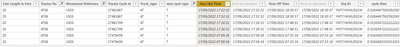Excel's result is as below,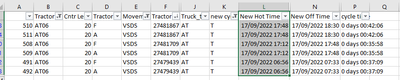Can see that the "New Hot Time" is not the same.

There are many more, but they are more or less similar to this case.

These are the steps I applied in PowerQuery for your information.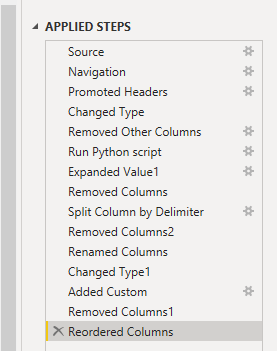Hope these help. Thank you very much.Super User

ah ok!   I didn't realize you were doing it that way.  Please can you share your m code, your power query code!  The answer may lie somewhere there.

If I took the time to answer your question and I came up with a solution, please mark my post as a solution and /or give kudos freely for the effort 🙂 Thank you!

Proud to be a Super User!Helper II

Sure, I believe it is the m-code.

Because from the "insert code sample" I can't find the m-code, let me upload it to the shared link below.Super User

sorry I was away.   I have gone through it. To me firstly it looks like the result in power bi is correct if your intention is to group by only  one tractor id as in 27481867, however if the purpose was to group both as in

 27481709 27481867

then I think potentially the way you have done it by adding the script as a separate step,  in might be where it it going wrong because its might only be joining on that relevant tractor id.

are you able to only run your python code and output the data from that one step in power query rather than the various steps and output it that way?  so as a new source just run the python code in full.. not after you have imported your file in.

If I took the time to answer your question and I came up with a solution, please mark my post as a solution and /or give kudos freely for the effort 🙂 Thank you!

Proud to be a Super User!Helper III went to "Transform Data" and cut all the additional steps after "expanded value" (right after running python script, have to expand it to view the table).

And then direct to Data page to see if the output has changed (though I don't think the output is different because basically all the steps being deleted have nothing to do with data itself.)

Applied filter to find the "problematic" task ID as before you and I did. It turns out to have the following filtered table. Compared with the Excel, the problem presists.You said

@vanessafvg wrote:

To me firstly it looks like the result in power bi is correct if your intention is to group by only  one tractor id as in 27481867 ......

May I know if you got a different answer from mine after running the python script?

Did you read the PowerBi file in the shared folder? I ain't sure if there might be some mistakes that I did wrong but I ain't aware of them.

Many thanks.Super User

yes that is what i used, and got exactly the same as you in both scenarios.

I created a few scenarios in the power bi file to test this tractor cycle id = 27481867

1. i created a table looking at source with the fields you used to get your date

2. created another table that looks at the output of the python from within m where the source was pulled in an m step separate from your script

3. then i created a  table that only looks at the script you created and i got the same result you get in pythonsee file attached.   however you still haven't highlighted which the correct answer is and i am sort of confused why python gets the answer it does as i dont see those values in either the source file directly or in the source that is imported into power bi.  However maybe I am missing something due to being a python novice.

If I took the time to answer your question and I came up with a solution, please mark my post as a solution and /or give kudos freely for the effort 🙂 Thank you!

Proud to be a Super User!Helper II

I am sorry that I missed your last response.

@vanessafvg wrote:the correct is "Only Run Python code" (the "New hot time" = 17/9/2022 17:48:31).

The reason of getting this value is due to the logic that I wrote in the python script. The logic is like this . It is basically doing sorting by "On chassis time" and try to amend the "hot time" based on whether the current row's "hot time" starts before the last row's "off chassis time". (It has been solved, but not by running python.) But I am still wondering what gives incosistent result.

Hope it helps.Super User

it definitely is an odd one i agree,  can't quite figure it out myself.  I think the steps between importing into power query and running the script might be uncessary and part of the issue.  Just running the script directly is clearly a better way to go.  How did you solve it?

If I took the time to answer your question and I came up with a solution, please mark my post as a solution and /or give kudos freely for the effort 🙂 Thank you!

Proud to be a Super User!Helper IIYou mean to delete the step "Changed Type" and "Removed Other Columns"?Super User

If you only run the python code you get the correct result, so instead of first ingesting the file with excel and doing some manipulations in power query and then inserting the python file, if you just run the python code (i adjusted it slightly the code to run directly in the source step of power query) then you get the correct result.    ie in the source step i run the python scriptso when ingest the file i just select  get data and select use python script rather than using excel to get datathis the code i ran in this step

# 'dataset' holds the input data for this script
import pandas as pd

def _truck_type(truck😞
if truck.find("AT") >= 0:
return "AT"
elif truck.find("T") >= 0:
return "MT"
else:
return "XT"

dataset = dataset.sort_values(by=["Tractor No","On Chasis Datetime"], ascending=[True,True])
dataset = dataset.reset_index(drop=True)

dataset["Truck_type"] = dataset["Tractor No"].map(_truck_type)
truck_type_list = ['AT', "MT"]

dataset = dataset[dataset["Truck_type"].isin(truck_type_list) == True]
dataset = dataset.reset_index(drop = True)

cycleID_dict = dataset["Tractor Cycle Id"].value_counts().to_dict()

dataset["Number of cycleID"] = dataset["Tractor Cycle Id"].map(cycleID_dict)

dataset["new cycle type"] = None

dataset["New Hot Time"] = dataset["Movement Hot Datetime"]

for i in range(dataset.shape):
dataset.loc[i, "new cycle type"] = "S" if dataset.loc[i,"Number of cycleID"] < 2 else "T"

for i in range(1, dataset.shape):
try:
if dataset.loc[i,"On Chasis Datetime"] <= dataset.loc[i, "Movement Hot Datetime"]:
if (dataset.loc[i, "Tractor No"] == dataset.loc[i-1, "Tractor No"]) and (dataset.loc[i, "new cycle type"] == "S") or (dataset.loc[i, "Tractor Cycle Type"] == "F" and dataset.loc[i, "new cycle type"] == "T"😞
dataset.loc[i, "New Hot Time"] = dataset.loc[i-1, "Off Chasis Datetime"]
elif (dataset.loc[i, "Tractor No"] == dataset.loc[i-2, "Tractor No"]) and (dataset.loc[i, "Tractor Cycle Type"] == "A" and dataset.loc[i, "new cycle type"] == "T"😞
dataset.loc[i, "New Hot Time"] = dataset.loc[i-2, "Off Chasis Datetime"]
except:
break

for i in range(1, dataset.shape):
try:
if dataset.loc[i, "New Hot Time"] <= dataset.loc[i-1, "New Hot Time"]:
if (dataset.loc[i, "Tractor No"] == dataset.loc[i-1, "Tractor No"]) and ((dataset.loc[i, "new cycle type"] == "S") or (dataset.loc[i, "Tractor Cycle Type"] == "F" and dataset.loc[i, "new cycle type"] == "T")):
if dataset.loc[i, "New Hot Time"] < dataset.loc[i-1, "Off Chasis Datetime"]:
dataset.loc[i, "New Hot Time"] = dataset.loc[i-1, "Off Chasis Datetime"]
elif (dataset.loc[i, "Tractor No"] == dataset.loc[i-2, "Tractor No"]) and (dataset.loc[i, "Tractor Cycle Type"] == "A" and dataset.loc[i, "new cycle type"] == "T"😞
if dataset.loc[i, "New Hot Time"] < dataset.loc[i-2, "Off Chasis Datetime"]:
dataset.loc[i, "New Hot Time"] = dataset.loc[i-1, "New Hot Time"]

except:
break

try:
if (dataset.loc[i, "Tractor No"] == dataset.loc[i-1, "Tractor No"]) and ((dataset.loc[i, "new cycle type"] == "S") or (dataset.loc[i, "Tractor Cycle Type"] == "F" and dataset.loc[i, "new cycle type"] == "T")):
if dataset.loc[i,"New Hot Time"] < dataset.loc[i-1,"Off Chasis Datetime"]:
dataset.loc[i,"New Hot Time"] = dataset.loc[i-1,"Off Chasis Datetime"]
elif (dataset.loc[i, "Tractor No"] == dataset.loc[i-2, "Tractor No"]) and (dataset.loc[i, "Tractor Cycle Type"] == "A" and dataset.loc[i, "new cycle type"] == "T"😞
if dataset.loc[i,"New Hot Time"] < dataset.loc[i-2,"Off Chasis Datetime"]:
dataset.loc[i, "New Hot Time"] = dataset.loc[i-1, "New Hot Time"]
except:
break

dataset['New Hot Time'] = dataset.groupby("Tractor Cycle Id")["New Hot Time"].transform('min')
dataset['New ht datetime'] = pd.to_datetime(dataset['New Hot Time']) #, format='%d-%b-%Y %H:%M:%S')
dataset['New Off Time'] = dataset.groupby("Tractor Cycle Id")["Off Chasis Datetime"].transform('max')
dataset['New ot datetime'] = pd.to_datetime(dataset['New Off Time']) #, format='%d-%b-%Y %H:%M:%S')

dataset['cycle time'] = dataset['New ot datetime']-dataset['New ht datetime']

dataset= dataset[dataset["Movement Reference"].isin(["VSDS","VSLD"]) == True]
print(dataset)

If I took the time to answer your question and I came up with a solution, please mark my post as a solution and /or give kudos freely for the effort 🙂 Thank you!

Proud to be a Super User!Helper II

@vanessafvg Haha, I finally found the root cause that made the difference. And can replicate the result of yours. As I copied the python script from python editor, where in it the variable is "df", while the PowerBI python editor forces us to use "dataset". I edited most of the "df" to "dataset".... but unfortunately, I overlooked one. It supposed to raise an error to me to warn me the variable is undefined yet, but I put the script in in "try" (which ignores any errors).

The following is to use MS Word to compare the script of mine and yours. And found out the small difference drives a big error on my side.So much thank and appreciation.Super User

oh cool!  glad that has been demysitified!! well done for figuring out why your original wasn't working.

If I took the time to answer your question and I came up with a solution, please mark my post as a solution and /or give kudos freely for the effort 🙂 Thank you!

Proud to be a Super User!Helper II

Brilliant! The result is correct!!!

But from my point of view, they are just doing the same thing, even the python script is almost identical (except importing data from python itself or from PowerBI). But turns out the are slightly different. Do you know what makes the difference? Or it is an internal bugs from PowerBI's python?Super User

fyi its changed all my ':' to faces

If I took the time to answer your question and I came up with a solution, please mark my post as a solution and /or give kudos freely for the effort 🙂 Thank you!

Proud to be a Super User!Announcements#### Exclusive opportunity for Women!

Join us for a free, hands-on Microsoft workshop led by women trainers for women where you will learn how to build a Dashboard in a Day!#### Power Platform Conference-Power BI and Fabric Sessions

Join us Oct 1 - 6 in Las Vegas for the Microsoft Power Platform Conference.Top Solution Authors
Top Kudoed Authors
Users online (3,512)